# AP Calculus AB Practice Test 40

### Test Information8 questions24 minutes

Calculator Allowed

1. The slope of the line tangent to the curve y = (arctan (ln x))2 at x = 2 is

2. Using the left rectangular method and four subintervals of equal width, estimate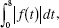where f is the function graphed below.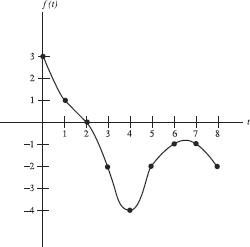3. Suppose f (3) = 2, f (3) = 5, and f (3) = -2. Then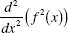at x = 3 is equal to

4. The velocity of a particle in motion along a line (for t ≥ 0) is v(t) = ln(2 -t2). Find the acceleration when the object is at rest.

5. Suppose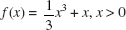and x is increasing. The value of x for which the rate of increase of f is 10 times the rate of increase of x is

6. The rate of change of the surface area, S, of a balloon is inversely proportional to the square of the surface area. Whichequation describes this relationship?

7. Two objects in motion from t = 0 to t = 3 seconds have positions x1(t) = cos(t2 + 1) and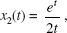respectively. How many times during the 3 seconds do the objects have the same velocity?

8. After t years, 50e -0.015t pounds of a deposit of a radioactive substance remain. The average amount per yearnot lost by radioactive decay during the second hundred years is